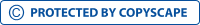Financial ManagementView larger

# Financial Management

546805

• High School
• 623

Short excerpt:

Find an estimate of the risk-free rate of interest (krf). To obtain this value, go to Bloomberg.com: Market Data and use the "U.S. 10-year Treasury" bond rate (middle column) as the risk-free rate. In addition, you also need a value for the market risk premium. Use an assumed market risk premium of 7.5%.ЊжUsing the information from the XYZ Stock Information document, record the following values:ЊжXYZ's beta (нЩ)Њж= 1.64 (60-month)XYZ's current annual dividend = \$ 0.80XYZ's 3-year dividend growth rate (g) = 8.2%Industry P/EЊж= 23.2XYZ's EPSЊж = \$ 4.87Here current dividend D = \$ 0.8 and the dividend growth rate = 8.2% therefore the expected dividend growth rate for next year will be D1 = 0.8 + (0.8*8.2%) = 0.8656%Now use the XYZ Stock Information to find XYZ's current stock quote (P).By buying this product you can collect up to 24 loyalty points. Your cart will total 24 loyalty points that can be converted into a voucher of \$0.24.

\$2.49